# Design and Implementation of an Improved Metaheuristic Algorithm for Maximum Power Point Tracking Algorithm Based on a PV Emulator and a Double-Stage Grid-Connected System

Design and Implementation of an Improved Metaheuristic Algorithm for Maximum Power Point Tracking Algorithm Based on a PV Emulator and a Double-Stage Grid-Connected System

Sami Meddour* | Djamel Rahem Patrice Wira Hichem Laib Ali Yahia Cherif | Ihssane Chtouki

Electrical Engineering and Automatic Laboratory (LGEA), Faculty of Sciences and Applied Sciences, Larbi Ben M'Hidi University, Oum El Bouaghi 04000, Algeria

Institute of Research in Computer Science, Mathematics, Automation and Signal, Haute Alsace University, 61 Rue Albert Camus, Mulhouse 68093, France

Department of Electrical Engineering, ERERA Research Team, ENSET, Mohammed V University, Avenue des Nations Unies, Rabat 10102, Morocco

Corresponding Author Email:
meddour.sami@yahoo.com
Page:
123-131
|
DOI:
https://doi.org/10.18280/ejee.240301
30 April 2022
|
Accepted:
22 June 2022
|
Published:
30 June 2022
| Citation

OPEN ACCESS

Abstract:

This research article proposes the optimization of a grid-connected photovoltaic system based on the PV emulator (PVE), the Boost converter, and a single-phase full-bridge inverter. The main objective is to track and extract the highest amount of power from the PV system working under variable solar irradiation while reducing losses and time response in each stage of the converter. As none, the main flaw of the classical maximum power point tracking algorithm (MPPT) is its performance. The control law design is based on a PI controller in the PVE, a meta heuristic whale optimization algorithm (WOA) in the MPPT, and a reduced switching finite set model predictive control (RS-FS-MPC) for the single-phase full-bridge inverter. The proposed control techniques inherit the property of robustness and successfully deal with the nonlinear behavior of the system. The performance validation and effectiveness of the proposed control techniques is supported by MATLAB/SIMULINK simulations and is also verified experimentally on various systems carried out under varying solar irradiation.

Keywords:

MPPT, converter, PV emulator, PV system, two level full-bridge inverter, PI controller, FS-MPC

1. Introduction

With the rapid increase in demand for power and the challenge of pollution, which has become a threat to everyone in the world. Especially in-developed countries that need more energy production to cover the growing demand in the industrial field . (SPV) technology is one of the most significant renewable resources that can be used to produce electricity .

The (SPV) system is envisaged to be a popular source of renewable energy, due to its significant advantages: low operational cost, with no noise, almost free maintenance, and low impact on the environment. Nevertheless, these systems still face a major obstacle due to their high investment cost, which is reflected in the cost per KWh of the energy produced and the efficiency of their energy conversion when compared with other renewable technologies. These drawbacks bring on increasing interest in the PV system and have led to many research papers in the last decade on various aspects, such as MPPT tracking speed, DC-DC converter topology enhancement, and the inverter controller efficiency. However, there is still a need to overcome this drawback.

A PVE will simulate the current and voltage characteristics of a photovoltaic panel under these varying conditions. Consistent electrical characteristics are reproduced, which allows easier analysis and system optimization with this PVE. Any type of PV panel characteristics with its scaled model can be emulated with dependent parameters, which will eliminate the need for costly PV panels. for this study, to determine the properties, the solar cell model may be simulated using a Newton-Raphson model circuit, and generalized models are given in the study .

Thus, many PVE control methods have been proposed with different algorithms [4-6]. In Ref. , a lookup table is used to store resistance, voltage, and current data at each point of the V-I characteristics, and a search algorithm is designed to locate the reference voltage at a particular load resistance. Some PVE are also developed using programmed power supplies with computer interface [8, 9].

## 1.png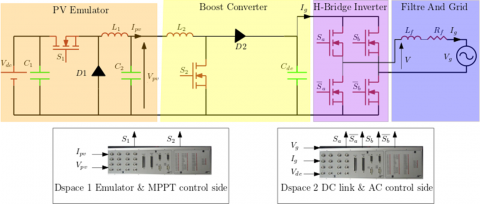Figure 1.  Scheme of the proposed cascaded control

There are several topologies for PVs, and they are classified according to the number of stages and whether they are connected or not to the utility grid [10, 11]. To date, single-phase grid-Connected PV inverters have been developed using either a single-stage or a two-stage topology. The single-stage topology providing the most reliable and cost-effective solution but with the operational limitation of the minimum PV voltage being greater than the peak ac grid voltage in order to operate. A two-stage approach, as illustrated in Figure 1, can function all over a wide range of PV voltage, maintaining adequate PV energy conversion.

The first stage of the two-stage grid-connected PV system employs a DC-DC converter, and at this stage, the PV power extraction is controlled. Moreover, the DC-DC converter is supposed to maintain the voltage of the PV side at a level suitable for normal operation on the grid connection. At this level of control, the MPPT is usually applied. Actually, the perturb and observe (P&O) [12, 13], and incremental conductance (INC) , approaches are the most commonly used strategies in the literature for MPPT extraction. However, both of them operate poorly in rapidly changing weather conditions. The MPPT techniques, founded on the meta-heuristic optimization technique, are becoming more and more popular in MPPT functionality in systems. Their main advantages are excellent performance based on evolutionary algorithms such as a proportional integral differential (PID) controller tuned by a genetic algorithm (GA) , and differential evaluation (DE) . On the other hand, swarm-based algorithms, such as practical swarm optimization [16, 17], and Grey wolf optimization (GW), Seyedali and Andrew  have presented a recent meta-heuristic optimization technique based on the hunting behaviour of a group of humpback whales. This foraging behaviour is called the "bubble-net feeding method". The quantitative performance developed and demonstrated in this paper supports the WOA algorithm's advantages over other approaches. They evaluated the WOA algorithm in 29 mathematical benchmark optimization problems and compared its performance to other commonly implemented heuristic techniques. This approach is very fast, which makes it suitable for mobile applications and can extract maximum power under varying irradiation.

The second stage of the two-stage system is the inverter stage. The subject of interest in this work is the single phase full bridge voltage source inverter. The most popular control strategies for it are voltage-oriented control (VOC) , and direct power control (DPC) , which are applied for such purposes. On the one hand, the VOC relies on the cascaded structure of the voltage and current loops, and its performance is mainly dependent on the proper tuning of the PI controllers. On the other hand, the DPC avoids the cascading structure of the VOC. However, it presents higher peak-to-peak ripples in the steady-state waveforms. More recently, predictive control techniques have become competitive with conventional controllers. They are simple to implement and straightforward. The approach minimizes the cost function, and the best switching state is implemented at the next sampling period. Control objectives and constraints can be easily implemented and included in the minimization function. The performance of FS-MPC has been demonstrated in a variety of applications, including electrical drives , wind turbines , energy management for smart and micro-grids , and PV applications .

In this paper, a PV emulator based on the PI controller (PI-PVE) with high performance is presented. Then, a metaheuristic algorithm (WOA) strategy for the PV system applications is developed to extract the MPP. On the AC side, a reduced switching FS-MPC has been implemented.

This paper is organized as follows: Section 2, presents the PV emulator design and modelling. Section 3, presents the proposed MPPT Technique. Section 4, demonstrate Grid side modelling and control. Section 5 illustrates the simulation results and their discussions. Section 6 shows the experimental test bench of the developed PI-PVE and the proposed MPPT (WOA) strategy and RS-FS-MPC for single-phase PV systems.

2. PV Emulator Design and Modelling

Simulation of conditions atmospherics in the laboratory is possible but the high cost of the complete system, also the dependence on climatic conditions, it turns out that it is necessary to find a way to emulate the operation of the photovoltaic panel with the possibility to simulate the variations of certain conditions atmospheric.

The realization of the photovoltaic emulator consists of reproducing the characteristic P-V curve of a PV panel. This schematic can emulate any PV panel for different illuminations, temperatures and load variation.

An emulator is composed of three main parts, a PV model, a control strategy and the buck converter (Figure 2).

The PV model receives temperature, irradiance, and measured PV voltage values as inputs. PV voltage is selected based on the power converter's control type. The output of the PV model is used as a reference for the closed loop system with a PI controller in order to control the output current.

## 2.png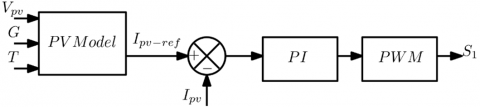Figure 2. The principal scheme of the PVE control strategy

2.1 Photovoltaic PV panel Model

The single-diode model is shown in Figure 3. This is the famous model of an equivalent electrical circuit for a photovoltaic cell. This model despite its simplicity gives very good results. Where the output current of the PV cell can be expressed according to many parameters, as indicated below.

## 3.png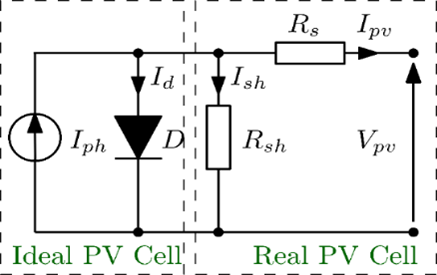Figure 3. Photovoltaic cell (single-diode)

${{I}_{pv}}\,=\,{{I}_{ph}}-{{I}_{D}}-{{I}_{rsh}}$      (1)

where, Iph is the Current generated by the incident light [A], ID is the diode current, expressed as:

${{I}_{D}}={{I}_{s}}\cdot ({{e}^{(\frac{q\cdot ({{V}_{pv}}+{{I}_{pv}}\cdot {{R}_{s}})}{n\cdot R\cdot T})}}-1)$      (2)

The current flowing through the shunt resistor is expressed by:

${{I}_{rsh}}=\frac{{{V}_{pv}}+{{I}_{pv}}\cdot {{R}_{s}}}{{{R}_{sh}}}$      (3)

where, Is is the diode saturation current [A], Rsh and Rs are respectively the parallel and series resistance. n the number of PV Cells connected in series, is the Boltzmann's constant [J/K], q the electron charge [C], T is the Kelvin temperature at standard test condition [K].

2.2 Buck converter overview

Figure 1 shows the buck converter with the switch S1, two capacitors C1, C2 and one inductor L1. The technique of operation adopted is the continuous conduction mode (CCM).

The transfer function of the buck converter is as follows:

${{G}_{s}}(s)=\,\frac{\overline{{{I}_{pv}}}(s)}{\overline{d}(s)}=\frac{{{V}_{dc}}}{R}\cdot \frac{1}{1+s\cdot \frac{L}{R}+{{s}^{2}}\cdot LC}$      (4)

Table 1. Electrical characteristics of Aavid Solar ASMS-220P PV module

 Characteristics Value (Unit) Isc 8.08 (A) Voc 36.8 (V) Impp 7.35 (A) Vmpp 30 (V) Pmpp 220.5 (W)

${{G}_{c}}(s)=\frac{{{k}_{p}}\cdot s+{{k}_{i}}}{s}$     (5)

where, Gs(s) and Gc(s) are the transfer functions of the buck converter and the compensator, respectively. The values of Kp and Ki of the PI controller are 0.0655 and 0.8321, respectively.

In the uncompensated system, the phase margin was 6.11 degrees. The PI parameters are calculated in terms of adding more stability and robustness to the system (in the compensated system, the phase margin is 58 degrees).

The Bode plot for the open-loop uncompensated and compensated systems is illustrated in Figure 4.

## 4.png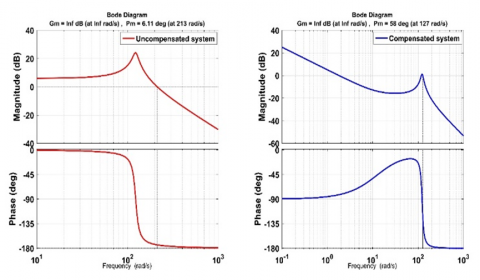Figure 4. Bode plot of the PI-PVE open-loop system

## 5.png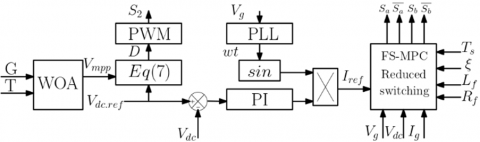Figure 5. The principal scheme of the grid-connected PV system control strategy

3. The Proposed MPPT Technique

3.1 Boost converter

The block diagram representation of proposed system is shown in Figure 1. The Boost converter is composed of switch S2, one inductor L2, and one diode D2.

The output and the input equation of the Boost Converter are as following:

${{V}_{dc}}=\frac{{{V}_{pv}}}{(1-D)}$     (6)

From Eq. (6), the duty cycle for the Boost converter is calculated directly from the following equation:

$D=1-\frac{{{V}_{mpp}}}{{{V}_{dc}}}$     (7)

3.2 Whale optimization algorithm

Humpback whales are one of the larger Mammals. The WOA is a recent meta-heuristic algorithm that mimicking the hunting behaviour of humpback whales. This impressive thing about them is that they Have large size, they typically reaching lengths of about 16 m and 30-tonne weight, furthermore are considering as highly intelligent animals.

## 6.pngFigure 6. Whale optimization algorithm 

Humpback whales operate an unusual and complex foraging behaviour called bubble netting that involves expelling air underwater to form a vertical cylinder-ring of bubbles around preys. Creating bubbles along a circle to force the prey to rise upon the surface (Figure 6).

In the three next subsections. The mathematical model of encircling prey, Search for prey and Spiral updating position is described.

3.2.1 Encircling prey

First, the humpback whales are distributed randomly in the search space, then they identify the location of prey and enclose them. The WOA algorithm considers that the current best whale solution is the nearest one to the prey target; in other words, it’s the closest one to the optimal problem solution. After the best hunting element is specified, the other search elements will try to update their positions toward the best one. The following equations represent this behaviour:

$D=\left| \overset{\to }{\mathop{C}}\,\overset{\to }{\mathop{{{X}^{*}}}}\,(t) \right.\left. -\overset{\to }{\mathop{X}}\,(t) \right|$     (8)

$\overset{\to }{\mathop{X}}\,(t+1)=\overset{\to }{\mathop{{{X}^{*}}}}\,(t)-\overset{\to }{\mathop{A}}\,\cdot \overset{\to }{\mathop{D}}\,$     (9)

where, $k$ is the current iteration, $X^{*}$ is the best whale position in the space research obtained in each iteration. $\vec{A}$ and $\vec{C}$ are calculated as follows:

$\overset{\to }{\mathop{A}}\,=2\cdot \overset{\to }{\mathop{a}}\,\cdot \overset{\to }{\mathop{r}}\,-\overset{\to }{\mathop{a}}\,$      (10)

$\overset{\to }{\mathop{C}}\,=2\cdot \overset{\to }{\mathop{r}}\,$     (11)

where, a is a linearly decreasing coefficient from 2 to 0 over the iteration (exploration and exploitation phase), and r is a random number [0, 1].

3.2.2 Search for prey

Encircling prey is very equivalent to searching for prey, but instead of using X, a randomly selected candidate, Xrand, is used in searching for prey. The process is described by the equation below:

$\overset{\to }{\mathop{D}}\,=\left| \overset{\to }{\mathop{C}}\,\overset{\to }{\mathop{\cdot {{X}_{rand}}}}\,(t)-\left. \overset{\to }{\mathop{X}}\, \right| \right.$     (12)

$\overset{\to }{\mathop{X}}\,(t+1)={{\overset{\to }{\mathop{X}}\,}_{rand}}(t)-\overset{\to }{\mathop{A}}\,\cdot \overset{\to }{\mathop{D}}\,$     (13)

WOA could indeed perform a global search by searching for prey during the exploration phase.

3.2.3 Spiral updating position

However during WOA's exploitation phase, encircling prey and spiral updating position strategies are included. Individuals' positions are changed by the spiral updating position to mimic the helix-shaped movement of humpback whales as follows:

$\overset{\to }{\mathop{X}}\,(t+1)={{D}^{'}}\cdot {{e}^{b\cdot l}}\cdot \cos (2\cdot \pi \cdot l)+\overset{\to }{\mathop{{{X}^{*}}}}\,(t)$      (14)

where, $D^{\prime}=\left|\vec{X}^{*}-\vec{X}(t)\right|$ describe the distance of the ith element to the best solution obtained, b defining the shape of the logarithmic spiral, l is a random number in [-1, 1].

Assuming a 50% chance of selecting either the shrinking encircling mechanism or the spiral model to update the position of whales throughout optimization, in order to force the search agent to move far away from reference whale. Where p is a random integer between 0 and 1.

 Algorithm (WOA algorithm). 1. Initialisation Xi (i = 1, 2, ..., NP) 2. Evaluate the fitness function for each whale in Xi 3. X* = the best whale (prey) 4. while The halting criterion is not satisfied do 5.     for i = 1 to NP do 6.           Update a, A, C, l and P; 7.        for j = 1 to n do 8.           if P < 0.5 then 9.              if (|A| < 1) 10.               D = | C. X* - Xi | 11.               Xi(j) = X* (j) - A.D 12.            else if (|A| >= 1) 13.               Select a random whale Xrand 14.               D = | C. Xrand - Xi | 15.               Xi(j) = Xrand(j) - A.D 16.            end if 17.         else if P >= 0.5 then 18.           D′ = |X* - Xi | 19.           Xi(j) = D′ . ebl. cos(2πl) + X* 20.         end if 21.       end for 22.       Evaluate the fitness for Xi 23.    end for 24. end while
4. Grid Side Modelling and Control

4.1 Single-phase full bridge two-level inverter connected to the grid model

Figure 1 shows the single-phase full-bridge inverter, where there are four switches: commutating in complementary for each $\operatorname{arm}\left(S_{a}, \bar{S}_{\mathrm{a}}\right)$ and $\left(S_{b}, \bar{S}_{\mathrm{b}}\right)$. The grid side is connected to the middle points between $S_{a}$ and $\bar{S}_{\mathrm{a}}$ in left-hand arm and $\mathrm{S}_{\mathrm{b}}$ and $\bar{S}_{\mathrm{b}}$ in the right-hand arm, respectively. It can generate an output voltage of $\left(+\mathrm{V}_{\mathrm{dc}}, 0,-\mathrm{V}_{\mathrm{dc}}\right)$, from the possible switching combination as shown in Table 2. In furthermore, four diodes, are used to provide paths for the load current that is driven by the stored energy in the ivad inductor. $T_{s}$ is the symbol for a cycle's period.

Table 2. Switching state and inverter output voltage

 Switching Vector Switching State Output Voltage Q1 Q2 Q3 Q4 V1 1 0 0 1 +Vdc V2 1 0 1 0 0 V3 0 1 1 0 -Vdc V4 0 1 0 1 0

The output inverter voltage V(t) is related to the DC bus-link voltage Vdc by the following equation:

$V(t)={{V}_{dc}}\cdot ({{S}_{a}}(t)\cdot \overline{{{S}_{b}}}(t)-{{S}_{b}}(t)\cdot \overline{{{S}_{a}}}(t))$     (15)

According to Figure 1, the differential equation of the single-phase full-bridge grid connected inverter is below:

$V={{L}_{f}}\cdot \frac{{{d}^{{}}}{{I}_{g}}}{{{d}_{{}}}t}+{{R}_{f}}\cdot {{I}_{g}}+{{V}_{g}}$      (16)

where, V and Ig are, respectively, the inverter output voltage and the grid current, and Lf and Rf are the inductors and the internal resistance of it, respectively.

4.2 Finite set model predictive control

The main idea of the FS-MPC strategy used in this paper is to predict the next step of the grid-connected current for every possible switching state combinations based on the discrete-time model of the single-phase inverter (Figure 7).

Theory to predict the future value of the grid-connected current the Euler approximation formula can be used for a sampling time Ts at the same sample k.

$\frac{d{{I}_{g}}}{{{d}_{{}}}t}=\frac{{{I}_{g}}\cdot (k+1)-{{I}_{g}}(k)}{{{T}_{s}}}$      (17)

The predicted grid current is calculated by applying Eq. (16) formula to the Eq. (17):

${{I}_{g}}(k+1)=(1-\frac{{{R}_{f}}{{T}_{s}}}{{{L}_{f}}})\cdot {{I}_{g}}(k)+\frac{{{T}_{s}}}{{{L}_{s}}}(V(k)-{{V}_{g}})$     (18)

Cost function: According to the full bridge model, RS-FS-MPC will predict the future behaviour of the output grid current for each of all voltage levels at the next sampling time. Each one of the four-state predictions is evaluated using the cost function equation. The switching state that minimizes the error between the grid-connected current and the reference current has been chosen as the optimal state and it will be applied in the next sampling period.

$f=\left| I_{p}^{g} \right.-\left. I_{ref}^{g} \right|+\xi \cdot \alpha$      (19)

Within a single period, ξ α is the term responsible for reducing the switching number in the inverter within a single period by adding the states that changed. Therefore, reduce the losses and enhance reliability and lifetime for the inverter by using the following equation.

$\alpha =\sum\limits_{i=1}^{4}{({{S}_{i}}(k+1)-{{S}_{i}}(k)})$      (20)

In the grid-connected converter, the DC-link is fixed to a reference value to guaranty the good operation of the single-phase inverter. For that a PI controller is implemented to adjust the DC Link voltage.

Note that the synchronization with the grid voltage is accomplished with a phase-locked loop (PLL).

where, w is the position of the voltage vector V estimated with a phase locked loop (PLL). In addition, grid-synchronised Iref is the product of sin(wt) and the output of the DC link controller, as shown in Figure 5.

## 7.pngFigure 7. Scheme of the RS-FS-MPC

5. Simulation Results and Discussions

To investigate the feasibility of the proposed topology of the PV system control, the PI-PVE, the MPPT controller, and the RS-FS-MPC control strategy are done in the MATLAB/SIMULINK environment. The PV panel parameters are indicated in Table 1 and the grid parameters in Table 3.

Table 3. Electrical characteristics of proposed PV system

 Characteristics Value (Unit) sampling Time Ts 1e-6 (S) filter inductor Lf 25 (mH) filter resistor Rf 0.1 (Ω) grid voltage Vg 50 (V) buck converter capacitor 1100 (µF) boost converter capacitor 1100 (µF) buck converter inductor 24 (mH) boost converter inductor 24 (mH)

To demonstrate the effectiveness of the proposed technique, the weather settings begin with a fixed irradiation and temperature at 1000 W/m2, 25°C, respectively. As illustrated in Figure 8, it is clear that the proposed algorithm reached the MPP in less than 5 ms. Furthermore, the proposed MPPT technique is stable and gives a perfectly steady-state response on clear days. Besides, dynamic insolation is performed, with 1000 W/m2 at the bagging and is reduced to 800 W/m2, then to 600 W/m2, and finally raised again slowly to 1000 W/m2, as illustrated in Figure 9. It can be shown that regardless of the irradiation disrupt variation, the program continues to quickly achieve the MPP within a short period of time using the proposed control strategy.

Grid voltage single-phase waveforms, grid measurement current, and the grid reference current are shown in Figure 11. It has been observed that the path to the new reference is quick and smooth, with a small overshoot, and that the grid voltage is in phase with the grid current. The THD current is 1.7%, and the power factor is nearly 1, As a result, the grid current is of good quality. A variation in climatic conditions is needed to evaluate the current controller's dynamic performance. The result of the performance is illustrated in Figure 10.

Figure 12, demonstrates the DC link voltage $(V d c)$ and its reference, and it confirms that the PI regulator works very well because the $V d c$ follows its reference very well.

Figure 13, shows the active energy injected into the grid through the RS-FS-MPC technique as well as the compensation of the reactive energy with a value of 0 VAR. For a sampling time of 10-6 S.

Figure 14, illustrates the reduction of the commutation number of the single-phase inverter, which is accomplished to save the converter transistor and keep the THD current below an acceptable rate. Concerning the second term of Eq. (20), it appears that it is possible to allow the inverter to work within an acceptable commutation number without impacting the existing THD. For the presented system, it is noticed that when the control strategy is performed without a weighting coefficient (λ=0). The THD current is nearly 1.67% with 400 commutations, at the same time, when the weighting coefficient is fixed to (ξ=0.02), the THD current is raised to 2.1 % with a drop of the commutation number from 400 to 187, and each time ξ is raised, the commutation drops and the THD current raised also.

## 8.png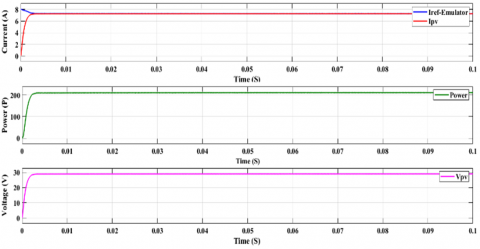Figure 8. Reference emulator current and PV measurement current, PV power, PV voltage at MPP

## 9.png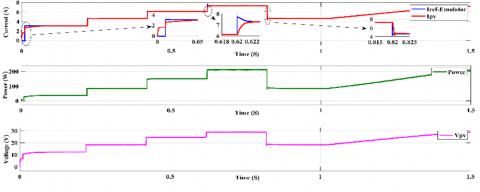Figure 9. Dynamic insolation, reference PV emulator current and PV measurement current, PV power, PV voltage at MPP

## 10.png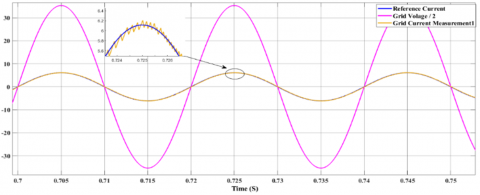Figure 10. Grid current measurement in dynamic irradiation

## 11.png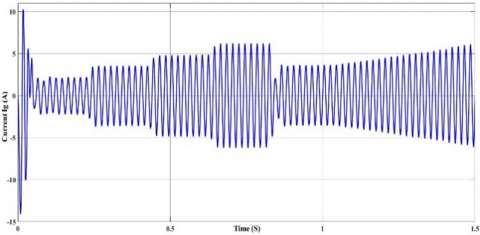Figure 11. Grid current with the grid current measurement, grid voltage

## 12.png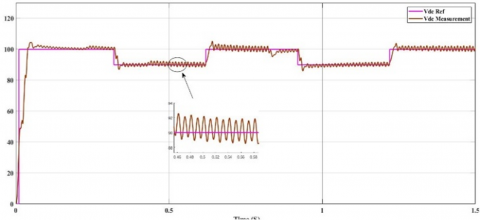Figure 12. DC regulated voltage control using a PI regulator

## 13.pngFigure 13. Active power transferred to the grid using FS-MPC technique

## 14.png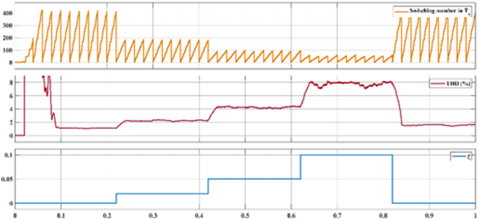Figure 14. Performance of the RS-FS-MPC, reducing of commutations number under 1000 kW/m2

6. Experimental Results

The experimental setup was established on a SEMIKRON IGBT (750 V/30A), one for each converter (Buck, Boost, and the inverter). The PVE has a power rating of 220 watts. The two converters and the inverter are connected in a cascade. The experimental setup is shown in Figure 15, where two DSpace were used as controllers to implement the proposed system; one DSpace for the PI control (PVE) and WOA (MPPT technique), which can be seen in Figure 15a, and the other to ensure the PI control (DC link regulation) and the grid side control (RS-FS-MPC technique), which can be seen in Figure 15b. The current and voltage are measured using LA-25 and LV-25 sensors, respectively.

Experimental results for the P-V curve operation for different irradiation levels of the PI-PVE control strategy are shown in Figures 16, 17, and 18. Experimental results for the dynamic performance of the reference current and the measured current of the PVE are demonstrated in Figure 19. It is seen that the performance in terms of speed tracking and ripple is very good.

In Figure 20, the dynamic performance of the proposed meta-heuristic WOA is shown in terms of track/extract power and speed response. The test starts with an irradiation of 1000 W/m2 with a fixed power of 212 watts. The value was abruptly changed to 800 W/m2. The algorithm's response time was less than 30 ms, and the power was decreased to 170 watts. After another reduction in irradiation to 600 W/m2, the power falls to 142 watts in less than 25 ms. After that, a sudden increase in irradiation is applied another time to test the algorithm in extreme conditions. This causes an increase in power and returns to the starting power in less than 60 ms. The recovery time after the solar variation was very high. And to minimize the number of calculations and avoid running the algorithm each time to calculate the same calculations for the same climatic conditions, to obtain MPP voltage and current, a lookup table was developed offline and filled in with all the MPP parameters.

The experimental test in Figure 21a shows that the grid current waveform is sine wave and perfectly in phase with the grid voltage. Figure 21b displays the performance of the RS-FS-MPC under irradiance variations and shows the grid current following the reference current with absolute accuracy.

Figure 22, depicts the system's strong dynamic performance to rapid changes in irradiance where active and reactive power are transmitted to the grid. Finally, the DC-link voltage regulation provides better performance, with a capacitor voltage variation of less than 2 V, which is nearly 2% of the overall DC-link voltage.

## 15a.png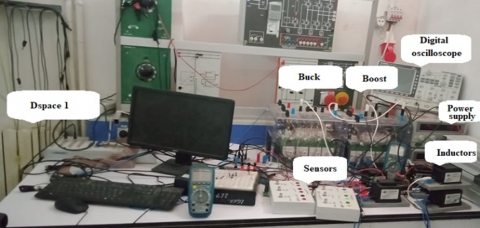(a)

## 15b.png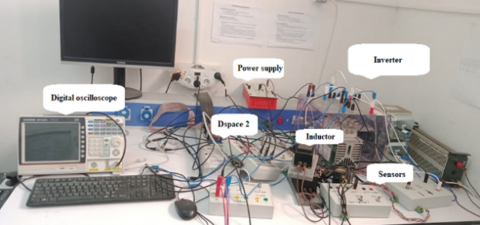(b)

Figure 15. Experimental bench

## 16.png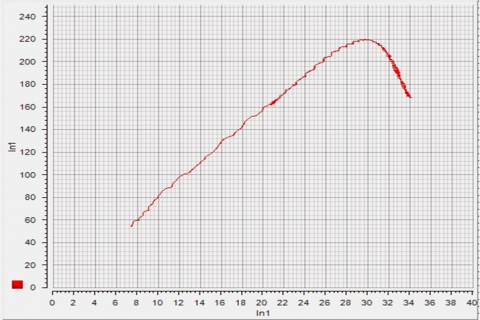Figure 16 Experimental P-V response for 1000 W/m2 25°C

## 17.png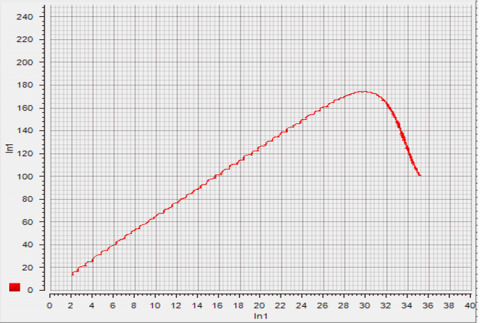Figure 17. Experimental P-V response for 800 W/m2 25°C

## 18.pngFigure 18. Experimental P-V response for 600 W/m2 25°C

## 19.pngFigure 19. Performances of the PV emulator, reference current and measurement current

## 20.png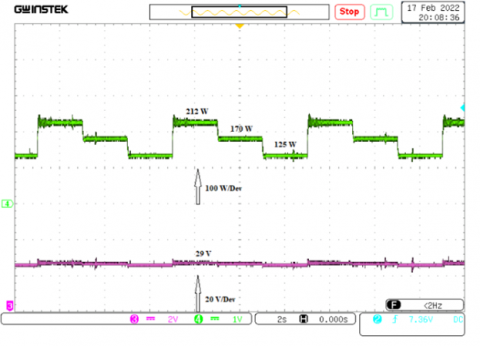Figure 20. Performances of the MPPT, Power extracted and grid voltage

## 21a.png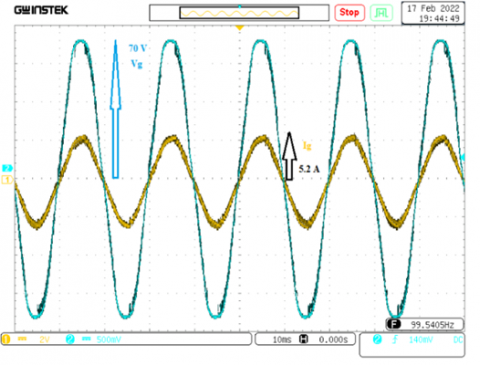(a)

## 21b.png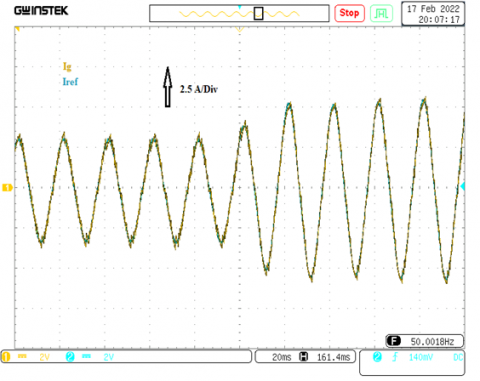(b)

Figure 21. Performance of the RS-FS-MPC under irradiance variations (Iref, Ig)

## 22.png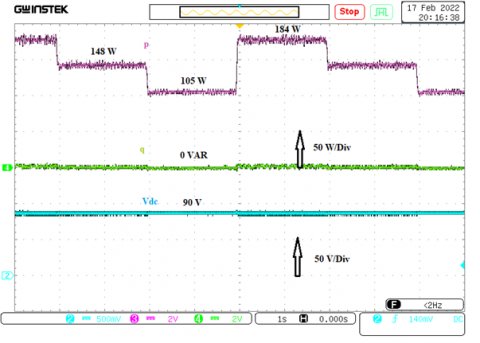Figure 22. Performance under irradiance variations. Active power (p), reactive power (q), DC bus voltage (Vdc)

7. Conclusions

In this paper, the principle of equivalent modelling for PV systems was presented, and the control strategy of the grid-connected PV system was revealed in detail, including PVE, MPPT control, and the inverter. The main role of all proposed systems is to track the MPP of a photovoltaic system and supply the utility grid with performance. The results obtained have testified to the effectiveness of the proposed controller.

References

 Ram, J.P., Manghani, H., Pillai, D.S., Babu, T.S., Miyatake, M., Rajasekar, N. (2018). Analysis on solar PV emulators: A review. Renewable and Sustainable Energy Reviews, 81: 149-160. https://doi.org/10.1016/j.rser.2017.07.039

 Murdock, Hannah E., Gibb, Duncan, Andre, Thomas, Sawin, Janet L., Brown, Adam, Ranalder, Lea, Andre, Thomas, Brown, Adam, Collier, Ute, Dent, Christopher, Epp, Baerbel, Hareesh Kumar, Chetna, Joubert, Fanny, Kamara, Ron, Ledanois, Nathalie, Levin, Rachele, Skeen, Jonathan, Sverrisson, Freyr, Wright, Glen, Passaro, Fabio, Guerra, Flavia, Dwi Sastriani, Ni Made, Yaqoob, Hend, Gicquel, Stefanie, Hamirwasia, Vibhushree, Kifukwe, Gwamaka, Yuan-Perrin, Yu, Mayer, Tammy, Williamson, Laura E., Budiman, Andreas, Chen, Olivia, Findlay, Katherine, Harris, Alyssa, Jones-Langley, Jessica, Urbani, Florencia, Mastny, Lisa, & Brumer, Leah (2021). Renewables 2021-Global status report (INIS-FR--21-0788). France. ISBN 978-3-948393-03-8.

 Reis, L.R.D., Camacho, J.R., Novacki, D.F. (2017). The Newton Raphson method in the extraction of parameters of PV modules. In International Conference on Renewable Energies and Power Quality, pp. 634-639. https://doi.org/10.24084/repqj15.416

 Binduhewa, P.J., Barnes, M. (2013). Photovoltaic emulator. In 2013 IEEE 8th International Conference on Industrial and Information Systems, pp. 519-524. https://doi.org/10.1109/ICIInfS.2013.6732038

 Nagayoshi, H., Orio, S., Kono, Y., Nakajima, H. (2002). Novel PV array/module IV curve simulator circuit. In Conference Record of the Twenty-Ninth IEEE Photovoltaic Specialists Conference, pp. 1535-1538. https://doi.org/10.1109/PVSC.2002.1190904

 Agrawal, J., Aware, M. (2012). Photovoltaic system emulator. In 2012 IEEE International Conference on Power Electronics, Drives and Energy Systems (PEDES), pp. 1-6. https://doi.org/10.1109/PEDES.2012.6484360

 Bhise, K., Pragallapati, N., Thale, S., Agarwal, V. (2012). Labview based emulation of photovoltaic array to study maximum power point tracking algorithms. In 2012 38th IEEE Photovoltaic Specialists Conference, pp. 002961-002966. https://doi.org/10.1109/PVSC.2012.6318206

 Dolan, D., Durago, J., Crowfoot, J. (2010). Simulation of a photovoltaic emulator. In North American Power Symposium, pp. 1-7. https://doi.org/10.1109/NAPS.2010.5618941

 Jiang, T., Putrus, G., McDonald, S., Conti, M., Li, B., Johnston, D. (2011). Generic photovoltaic system emulator based on lambert omega function. In 2011 46th International Universities' Power Engineering Conference (UPEC), pp. 1-5.

 Remache, S.E.I., Cherif, A.Y., Barra, K. (2019). Optimal cascaded predictive control for photovoltaic systems: application based on predictive emulator. IET Renewable Power Generation, 13(15): 2740-2751. https://doi.org/10.1049/iet-rpg.2019.0068

 Chtouki, I., Wira, P., Zazi, M., Cherif, A.Y., Meddour, S. (2020). A new control stratum applied to two adaptation stages based on Adaline-type neuronal predictive control in a photovoltaic solar conversion chain. European Journal of Electrical Engineering, 22(4-5): 365-376. https://doi.org/10.18280/ejee.224-508

 Meddour, S., Rahem, D., Cherif, A. Y., Hachelfi, W., Hichem, L. (2019). A novel approach for PV system based on metaheuristic algorithm connected to the grid using FS-MPC controller. Energy Procedia, 162: 57-66. https://doi.org/10.1016/j.egypro.2019.04.007

 Chtouki, I., Wira, P., Zazi, M., Collicchio, B., Meddour, S. (2019). Design, implementation and comparison of several neural perturb and observe MPPT methods for photovoltaic systems. International Journal of Renewable Energy Research (IJRER), 9(2): 757-770. https://doi.org/10.20508/ijrer.v9i2.9293.g7645

 Kotla, R.W., Yarlagadda, S.R. (2020). Grid tied solar photovoltaic power plants with constant power injection maximum power point tracking algorithm. Journal Europeen des Systemes Automatises, 53(4): 567-573, https://doi.org/10.18280/jesa.530416

 Salih, H.W., Wang, S., Farhan, B.S. (2015). A novel GA-PI optimized controller for MPPT based PV in a hybrid PV-diesel power system. In 2015 5th International Conference on Electric Utility Deregulation and Restructuring and Power Technologies (DRPT), pp. 1288-1293. https://doi.org/10.1109/DRPT.2015.7432428

 Joisher, M., Singh, D., Taheri, S., Espinoza-Trejo, D. R., Pouresmaeil, E., Taheri, H. (2020). A hybrid evolutionary-based MPPT for photovoltaic systems under partial shading conditions. IEEE Access, 8: 38481-38492. https://doi.org/10.1109/ACCESS.2020.2975742

 Hayder, W., Abid, A., Hamed, M.B., Ogliari, E., Sbita, L. (2021). Comparison of MPPT methods FLC & PSO for PV system under variable irradiance and temperature. In 2021 18th International Multi-Conference on Systems, Signals & Devices (SSD), pp. 1247-1251. https://doi.org/10.1109/SSD52085.2021.9429346

 Mirjalili, S., Lewis, A. (2016). The whale optimization algorithm. Advances in Engineering Software, 95: 51-67. https://doi.org/10.1016/j.advengsoft.2016.01.008

 Lang, B., Zhang, H., Sun, L., Wang, B., Zheng, X. (2019). Design of three phase grid-connected inverter based on grid-voltage oriented control. In 2019 Chinese Control Conference (CCC), pp. 6525-6530. https://doi.org/10.23919/ChiCC.2019.8865694

 Benbouhenni, H., Boudjema, Z., Belaidi, A. (2020). DPC based on ANFIS super-twisting sliding mode algorithm of a doubly-fed induction generator for wind energy system. Journal Européen des Systèmes Automatisés, 53(1): 69-80, https://doi.org/10.18280/jesa.530109

 Hamouda, N., Babes, B., Kahla, S., Soufi, Y. (2019). Real time implementation of grid connected wind energy systems: Predictive current controller. In 2019 1st International Conference on Sustainable Renewable Energy Systems and Applications (ICSRESA), pp. 1-6. https://doi.org/10.1109/ICSRESA49121.2019.9182526

 Rana, M.J., Abido, M.A. (2017). Energy management in DC microgrid with energy storage and model predictive controlled AC–DC converter. IET Generation, Transmission & Distribution, 11(15): 3694-3702. https://doi.org/10.1049/iet-gtd.2016.1934

 Ram, J.P., Manghani, H., Pillai, D.S., Babu, T.S., Miyatake, M., Rajasekar, N. (2018). Analysis on solar PV emulators: A review. Renewable and Sustainable Energy Reviews, 81: 149-160. https://doi.org/10.1109/ICRERA.2017.8191127